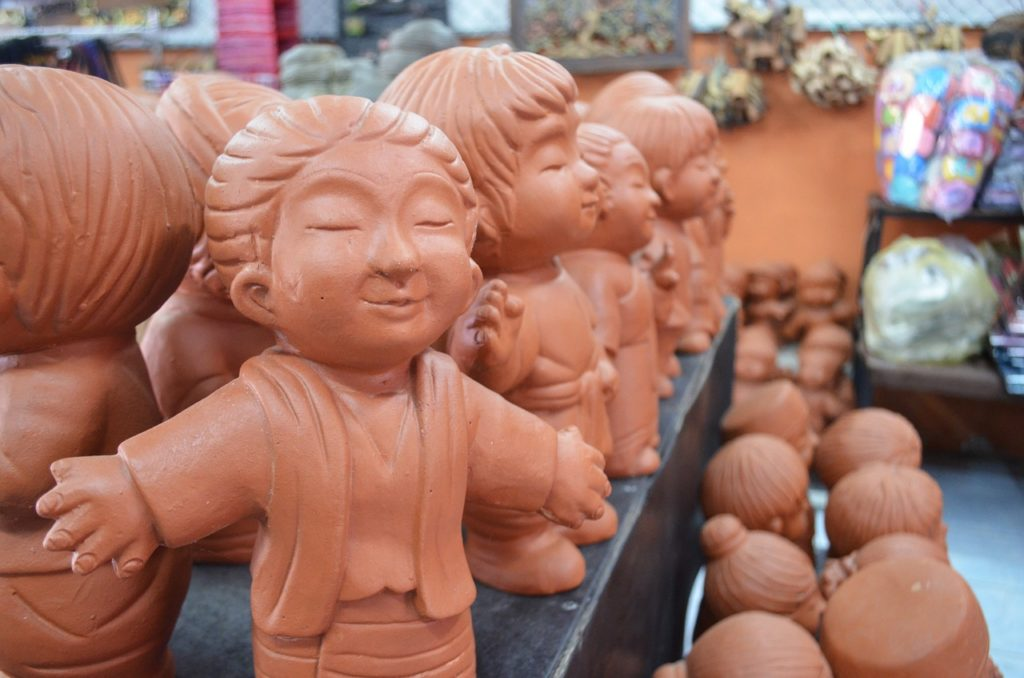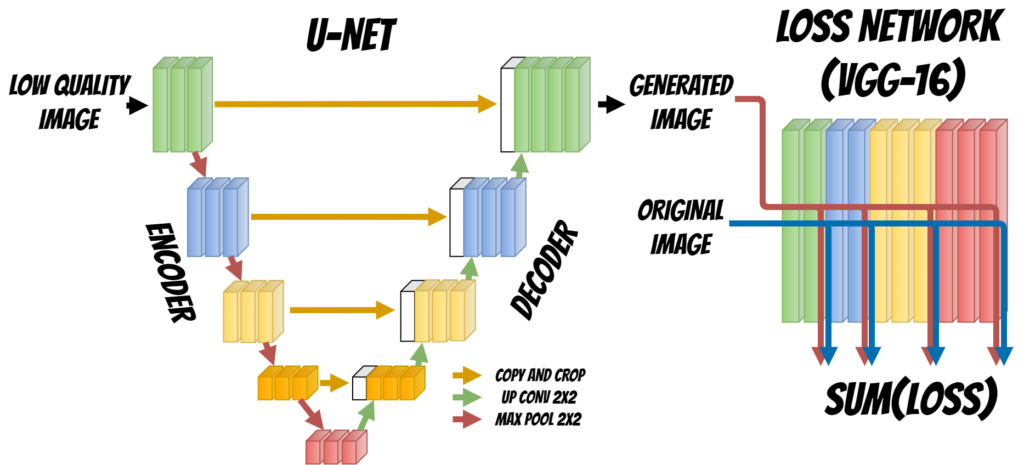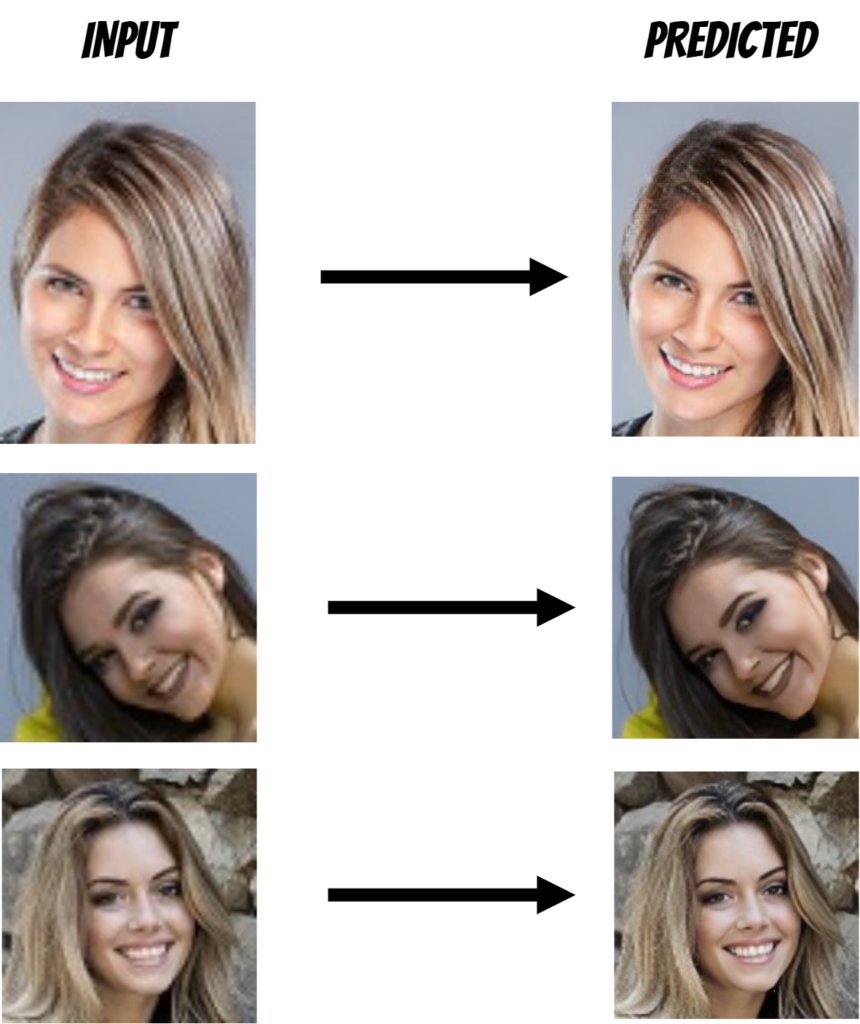# Unblur low quality face images with AIIn this article I will show how to improve the quality of blurred face images using artificial intelligence. For this purpose I will use neural networks and FastAI library (ver. 1)

The project code is available on my github: https://github.com/qooba/aiunblur You can also use ready docker image: https://hub.docker.com/repository/docker/qooba/aiunblur

I have based o lot on the fastai course thus I definitely recommend to go through it.

## Data

To train neural network how to rebuild the face images we need to provide the faces dataset which will show how low quality and blurred images should be reconstructed. Thus we need pairs of low and high quality images.

To prepare the data set we can use available fases dataset eg. FFHQ, Tufts Face Database, CelebA

We will treat the original images as a high resolution data and rescale them to prepare low resolution input:

``````import fastai
from fastai.vision import *
from fastai.callbacks import *
from fastai.utils.mem import *
from torchvision.models import vgg16_bn
from pathlib import Path

path = Path('/opt/notebooks/faces')
path_hr = path/'high_resolution'
path_lr = path/'small-96'

il = ImageList.from_folder(path_hr)

def resize_one(fn, i, path, size):
dest = path/fn.relative_to(path_hr)
dest.parent.mkdir(parents=True, exist_ok=True)
img = PIL.Image.open(fn)
targ_sz = resize_to(img, size, use_min=True)
img = img.resize(targ_sz, resample=PIL.Image.BILINEAR).convert('RGB')
img.save(dest, quality=60)

sets = [(path_lr, 96)]
for p,size in sets:
if not p.exists():
print(f"resizing to {size} into {p}")
parallel(partial(resize_one, path=p, size=size), il.items)
``````

Now we can create data bunch for training:

``````bs,size=32,128
arch = models.resnet34
src = ImageImageList.from_folder(path_lr).split_by_rand_pct(0.1, seed=42)

def get_data(bs,size):
data = (src.label_from_func(lambda x: path_hr/x.name)
.transform(get_transforms(max_zoom=2.), size=size, tfm_y=True)
.databunch(bs=bs,num_workers=0).normalize(imagenet_stats, do_y=True))

data.c = 3
return data

data = get_data(bs,size)
``````

## Training

In this solution we will use a neural network with UNET architecture.The UNET neural network contains two parts Encoder and Decoder which are used to reconstruct the face image. During the first stage Encoder fetch the input, extracts and aggregates the image features. At each stage the features maps are donwsampled. Then Decoder uses extracted features and tries to rebuild the image upsampling it at each decoding stage. Finally we get regenerated images.

Additionally we need to define the Loss Function which will tell the model if the image was rebuilt correctly and allow to train the model.

To do this we will use additional neural network VGG-16. We will put Generated image and Original image (which is our target) to the network input. Then will compare the features extracted for both images at selected layers and according to this calculated the loss.

Finally we will use Adam optmizer to minimize the loss and achieve better result.

``````def gram_matrix(x):
n,c,h,w = x.size()
x = x.view(n, c, -1)
return (x @ x.transpose(1,2))/(c*h*w)

base_loss = F.l1_loss

vgg_m = vgg16_bn(True).features.cuda().eval()

blocks = [i-1 for i,o in enumerate(children(vgg_m)) if isinstance(o,nn.MaxPool2d)]

class FeatureLoss(nn.Module):
def __init__(self, m_feat, layer_ids, layer_wgts):
super().__init__()
self.m_feat = m_feat
self.loss_features = [self.m_feat[i] for i in layer_ids]
self.hooks = hook_outputs(self.loss_features, detach=False)
self.wgts = layer_wgts
self.metric_names = ['pixel',] + [f'feat_{i}' for i in range(len(layer_ids))
] + [f'gram_{i}' for i in range(len(layer_ids))]

def make_features(self, x, clone=False):
self.m_feat(x)
return [(o.clone() if clone else o) for o in self.hooks.stored]

def forward(self, input, target):
out_feat = self.make_features(target, clone=True)
in_feat = self.make_features(input)
self.feat_losses = [base_loss(input,target)]
self.feat_losses += [base_loss(f_in, f_out)*w
for f_in, f_out, w in zip(in_feat, out_feat, self.wgts)]
self.feat_losses += [base_loss(gram_matrix(f_in), gram_matrix(f_out))*w**2 * 5e3
for f_in, f_out, w in zip(in_feat, out_feat, self.wgts)]
self.metrics = dict(zip(self.metric_names, self.feat_losses))
return sum(self.feat_losses)

def __del__(self): self.hooks.remove()

feat_loss = FeatureLoss(vgg_m, blocks[2:5], [5,15,2])

learn = unet_learner(data, arch, wd=wd, loss_func=feat_loss, callback_fns=LossMetrics,
blur=True, norm_type=NormType.Weight)

``````

## Results

After training we can use the model to regenerate the images:## Application

Finally we can export the model and create the drag and drop application which fix the face images in web application.The whole solution is packed into docker images thus you can simply start it using commands:

``````# with GPU
docker run -d --gpus all --rm -p 8000:8000 --name aiunblur qooba/aiunblur

# without GPU
docker run -d --rm -p 8000:8000 --name aiunblur qooba/aiunblur
``````

To use GPU additional nvidia drivers (included in the NVIDIA CUDA Toolkit) are needed.